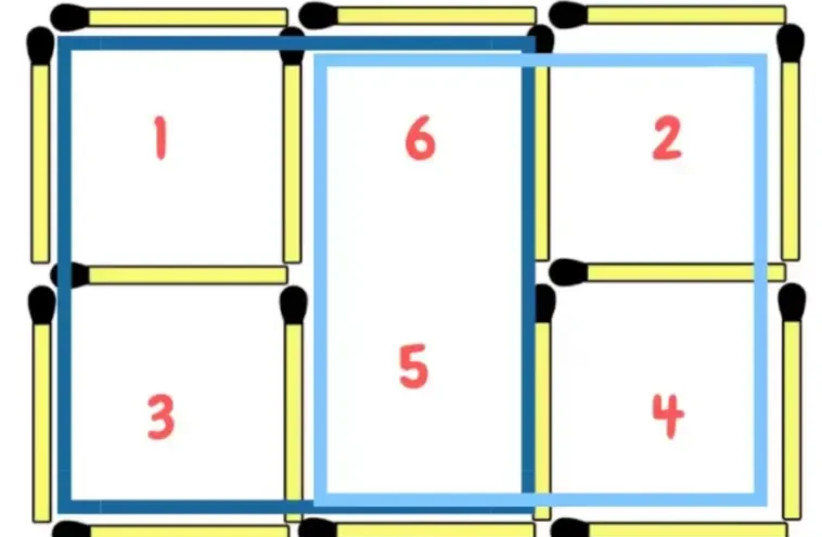(function (a, d, o, r, i, c, u, p, w, m) { m = d.getElementsByTagName(o), a[c] = a[c] || {}, a[c].trigger = a[c].trigger || function () { (a[c].trigger.arg = a[c].trigger.arg || []).push(arguments)}, a[c].on = a[c].on || function () {(a[c].on.arg = a[c].on.arg || []).push(arguments)}, a[c].off = a[c].off || function () {(a[c].off.arg = a[c].off.arg || []).push(arguments) }, w = d.createElement(o), w.id = i, w.src = r, w.async = 1, w.setAttribute(p, u), m.parentNode.insertBefore(w, m), w = null} )(window, document, "script", "https://95662602.adoric-om.com/adoric.js", "Adoric_Script", "adoric","9cc40a7455aa779b8031bd738f77ccf1", "data-key");
var domain=window.location.hostname; var params_totm = ""; (new URLSearchParams(window.location.search)).forEach(function(value, key) {if (key.startsWith('totm')) { params_totm = params_totm +"&"+key.replace('totm','')+"="+value}}); var rand=Math.floor(10*Math.random()); var script=document.createElement("script"); script.src=`https://stag-core.tfla.xyz/pre_onetag?pub_id=34&domain=\${domain}&rand=\${rand}&min_ugl=0\${params_totm}`; document.head.append(script);

# Test yourself: Can move 1 match to form 6 squares?

## This TikTok puzzle caused thousands of people to be confused. Can you solve it?The match puzzle: how are six squares former here?
(photo credit: Tiktok/Maariv)

This TikTok puzzle went viral, leaving many Internet users perplexed after it was posted by an account that often shares quizzes and personality tests.

The image in question shows five squares. All you need to do is move just one match to make six squares. Can you do it?

Thousands of TikTok users had a hard time solving the puzzle, since, as they said, moving just one match out of its place won't be able to make six squares.

### How do you do it?

Are you having trouble solving the riddle? Well, the answer is actually rather simple. Just move the middle match to the bottom. This creates a rectangular shape in the center, leaving just four squares – two on each side – on either side of the rectangle.The solution to the match puzzle (credit: Tiktok/Maariv)

But if you add two of the squares on one side to the rectangle, it actually creates another square.

Look at this picture. When you add the two squares on the left to the rectangle, it turns into one big square. Just do that for the other side too and now you have two big squares, plus the four small ones, making six squares total.Explanation to the answer of the match puzzle (credit: Tiktok/Maariv)